You are currently offline. Some features of the site may not work correctly.

# Rainbow matching

In the mathematical discipline of graph theory, a rainbow matching in an edge-colored graph is a matching in which all the edges have distinct colors… Expand
Wikipedia

## Papers overview

Semantic Scholar uses AI to extract papers important to this topic.
2017
2017
Aharoni and Berger conjectured that every bipartite graph which is the union of n matchings of size n + 1 contains a rainbow… Expand
•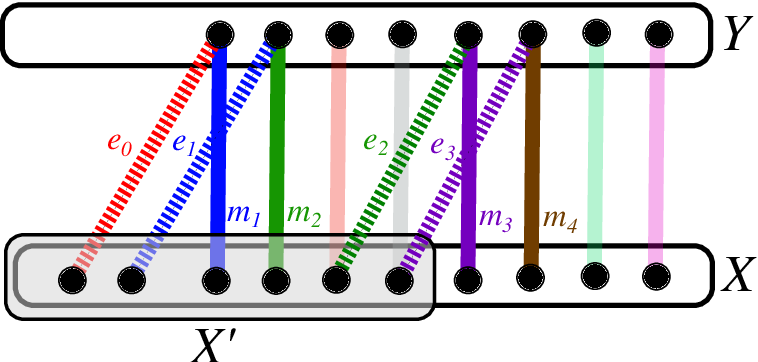Is this relevant?
2014
2014
• Australas. J Comb.
• 2014
• Corpus ID: 1991920
We show that there exists a bipartite graph containing n matchings of sizes mi n satisfying ∑ i mi = n 2 + n/2 − 1, such that the… Expand
Is this relevant?
2014
2014
• Electr. J. Comb.
• 2014
• Corpus ID: 6582393
Given a coloring of the edges of a multi-hypergraph, a rainbow $t$-matching is a collection of $t$ disjoint edges, each having a… Expand
Is this relevant?
2014
2014
• Theor. Comput. Sci.
• 2014
• Corpus ID: 10236511
A rainbow matching in an edge-colored graph is a matching whose edges have distinct colors. We address the complexity issue of… Expand
Is this relevant?
2013
2013
• 2013
• Corpus ID: 116747700
A recent conjecture of Aharoni, Charbit and Howard states that $n$ matchings, each of size $n+1$, in a bipartite graph have a… Expand
•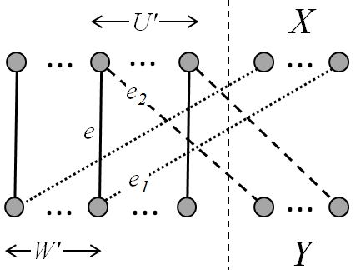•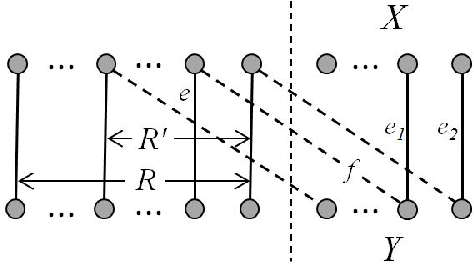•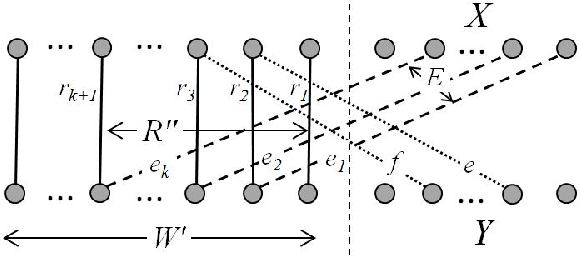•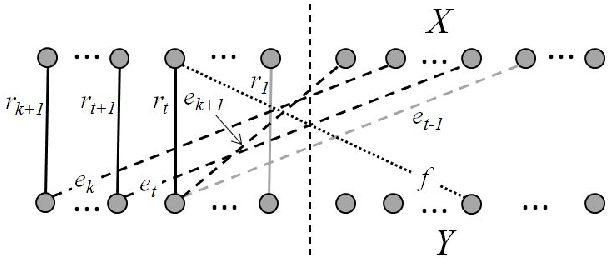Is this relevant?
2012
2012
• Comb. Probab. Comput.
• 2012
• Corpus ID: 15954215
A rainbow subgraph of an edge-coloured graph is a subgraph whose edges have distinct colours. The colour degree of a vertex v is… Expand
•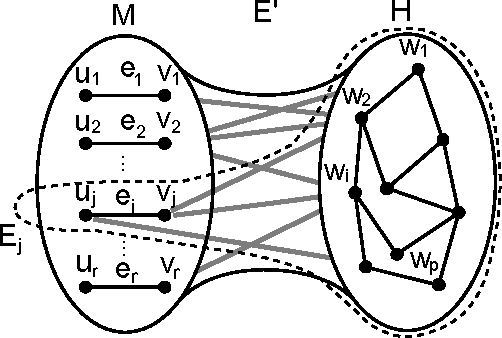•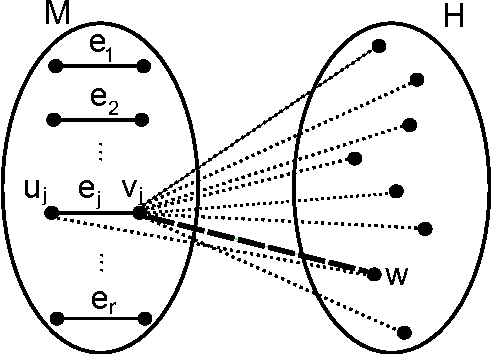•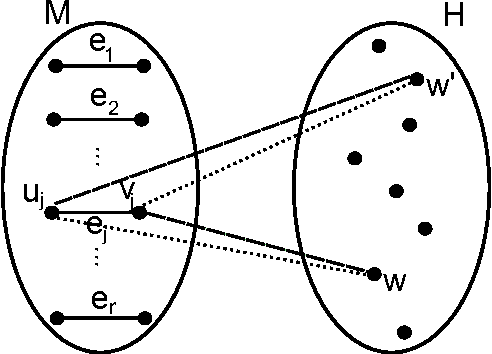Is this relevant?
2011
2011
Let $G$ be a properly edge colored graph. A rainbow matching of $G$ is a matching in which no two edges have the same color. Let… Expand
Is this relevant?
2010
2010
• Electr. J. Comb.
• 2010
• Corpus ID: 7721730
A rainbow subgraph of an edge-colored graph is a subgraph whose edges have distinct colors. The color degree of a vertex $v$ is… Expand
•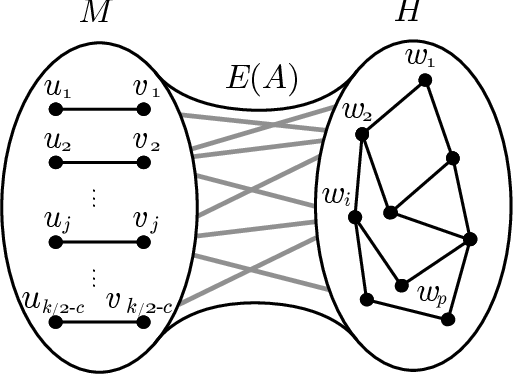Is this relevant?
Highly Cited
2009
Highly Cited
2009
• Electr. J. Comb.
• 2009
• Corpus ID: 2016279
Given a collection of matchings ${\cal M} = (M_1, M_2, \ldots, M_q)$ (repetitions allowed), a matching $M$ contained in $\bigcup… Expand Is this relevant? 2009 2009 • Electr. J. Comb. • 2009 • Corpus ID: 15320142 An$r$-edge-coloring of a graph is an assignment of$r$colors to the edges of the graph. An exactly$r\$-edge-coloring of a graph… Expand
•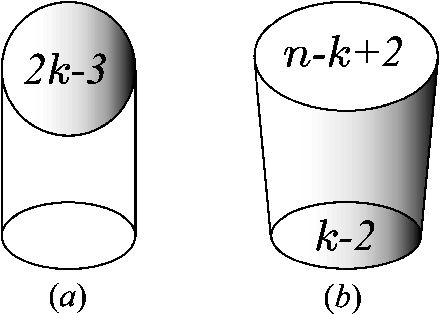•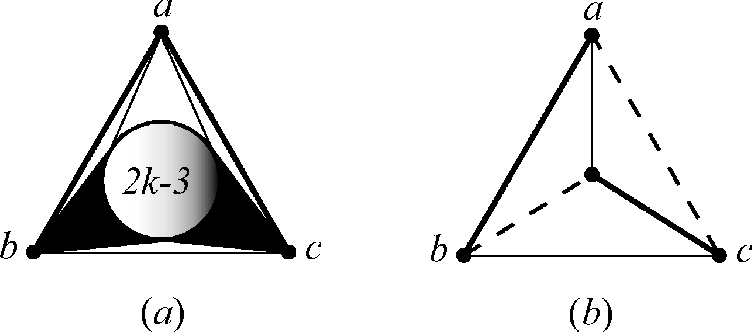•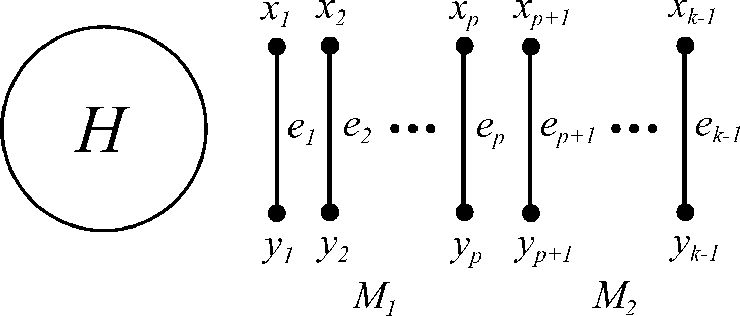•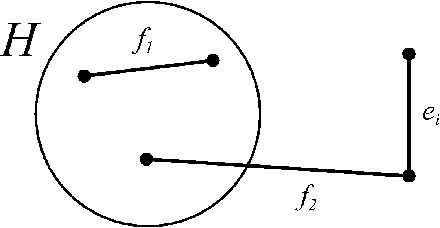•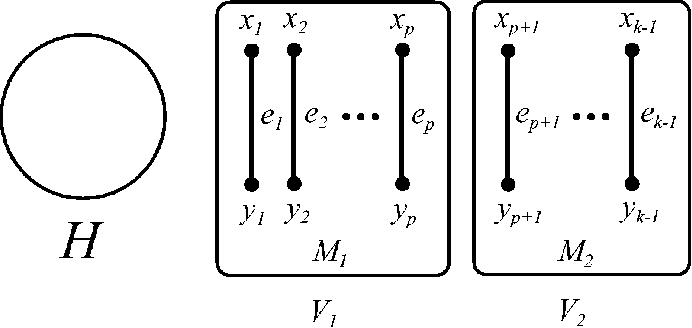Is this relevant?